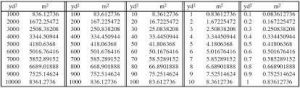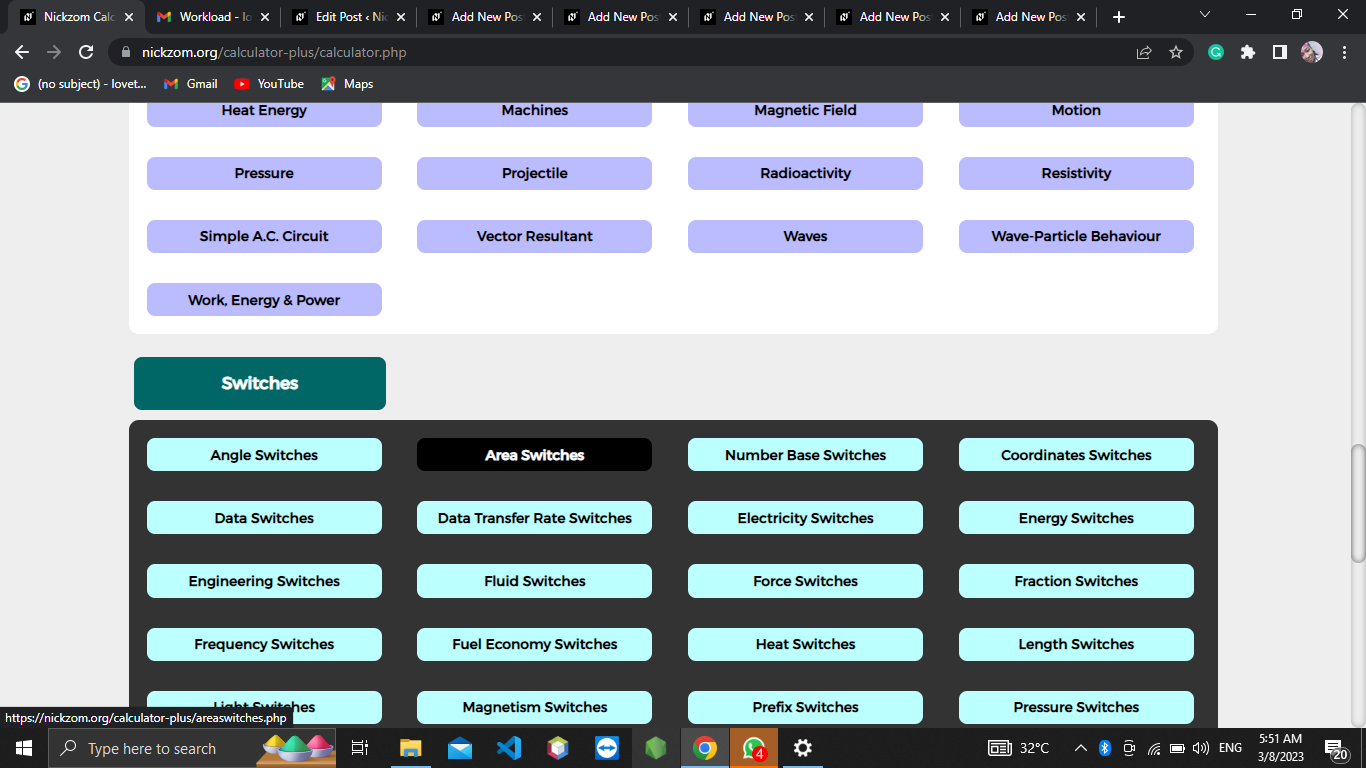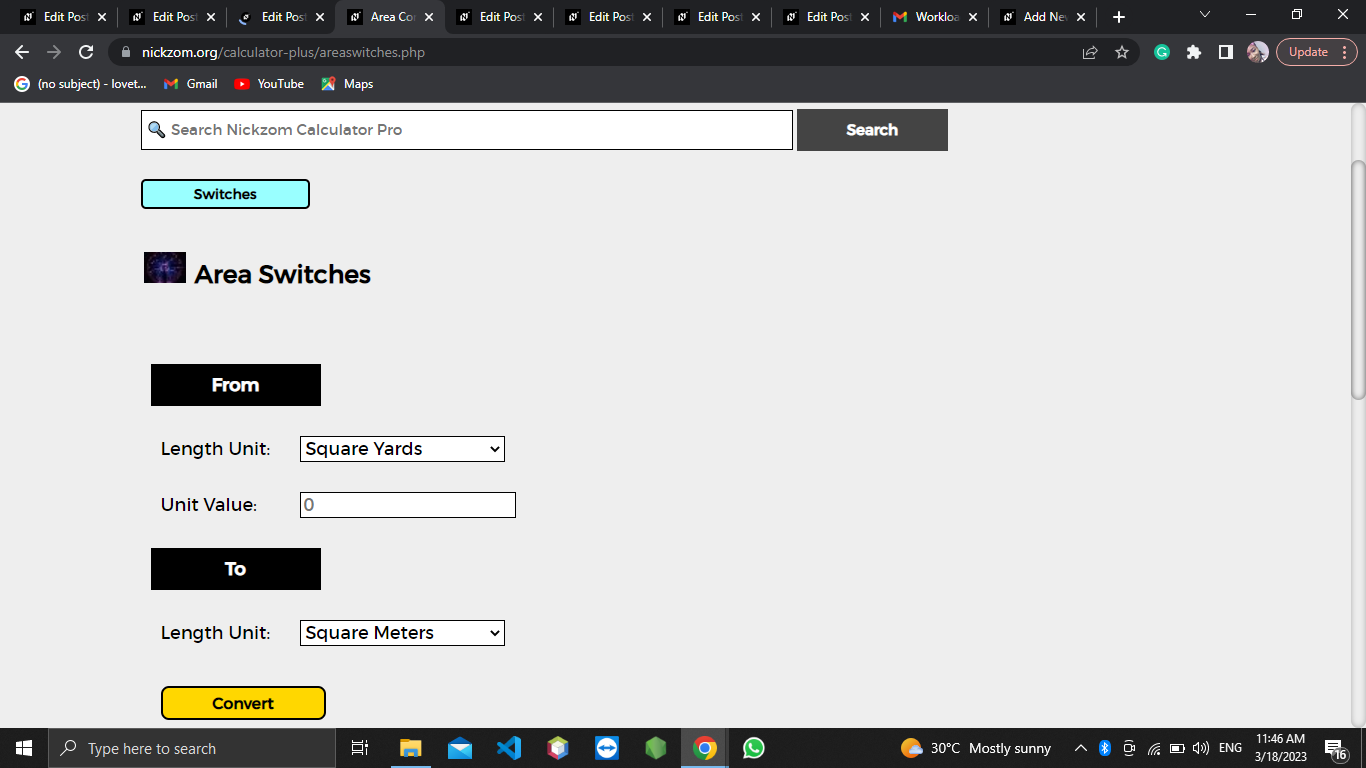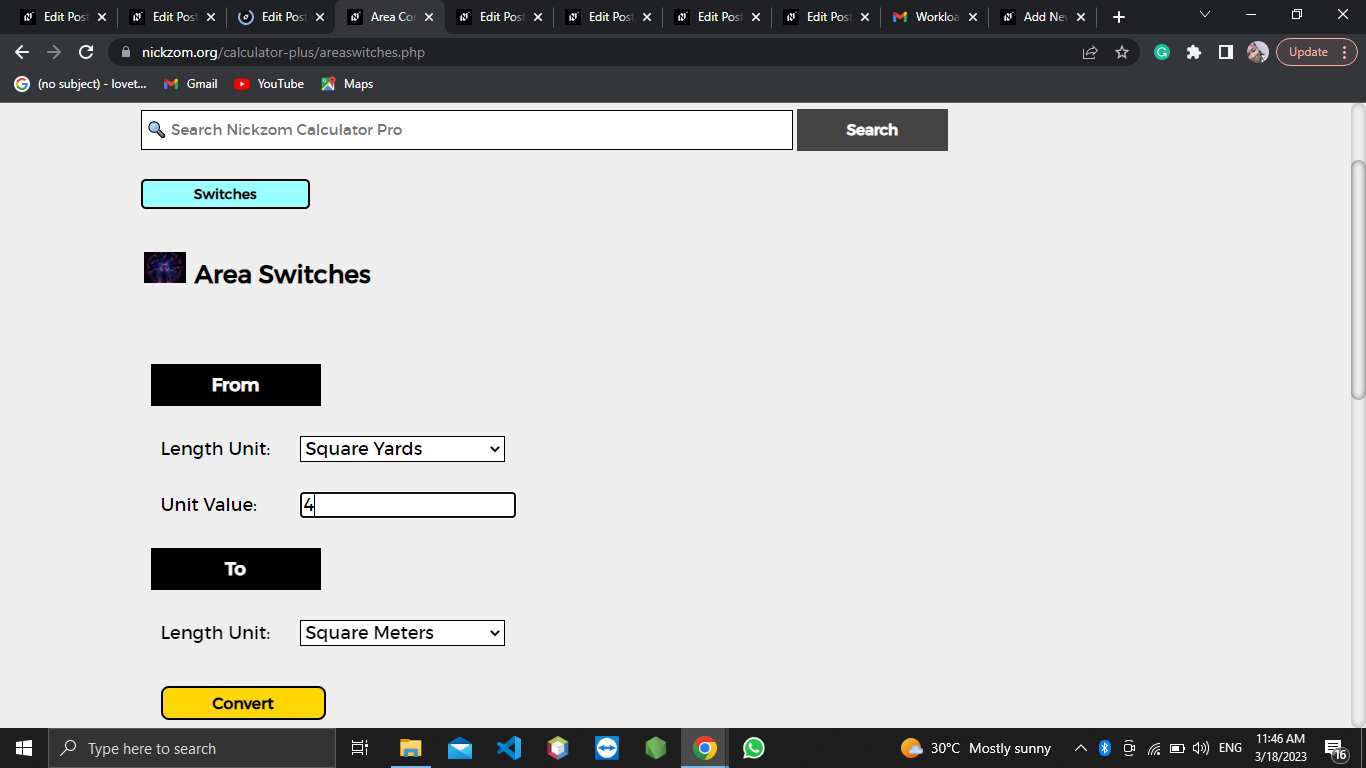# How to Convert Square Yards to Square Meters | Area Units

The image below represents square yards to square meters.To convert square yards to square meters, one essential parameter is needed and this parameter is Unit Value.

The formula for converting square yards to square meters:

1 Square Yard = 0.836127 Square Meters

Let’s solve an example;
Find the conversion of square yards to square meters when the unit value is 4.

1 Square Yard = 0.836127 Square Meters

Then,

4 Square Yards = (4 x 0.836127) Square Meters
4 Square Yards = 3.344508 Square Meters

Therefore, the square meter(s) is 3.344508.

Nickzom Calculator – The Calculator Encyclopedia is capable of converting square yards to square meters.

To get the answer and workings of square yards to square meters using the Nickzom Calculator – The Calculator Encyclopedia. First, you need to obtain the app.

You can get this app via any of these means:

You can also try the demo version via https://www.nickzom.org/calculator

Apple (Paid) – https://itunes.apple.com/us/app/nickzom-calculator/id1331162702?mt=8
Once, you have obtained the calculator encyclopedia app, proceed to the Calculator Map, then click on Area Units under Switches.The screenshot below displays the page or activity to enter your value, to get the answer for the conversion of square yards to square meters according to the respective parameter which is the Unit Value.Now, enter the value appropriately and accordingly for the parameter as required by the Unit Value is 4.Finally, Click on CalculateAs you can see from the screenshot above, Nickzom Calculator– The Calculator Encyclopedia solves the conversion of square yards to square meters and presents the formula, workings, and steps too.